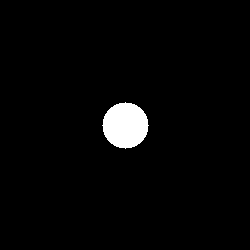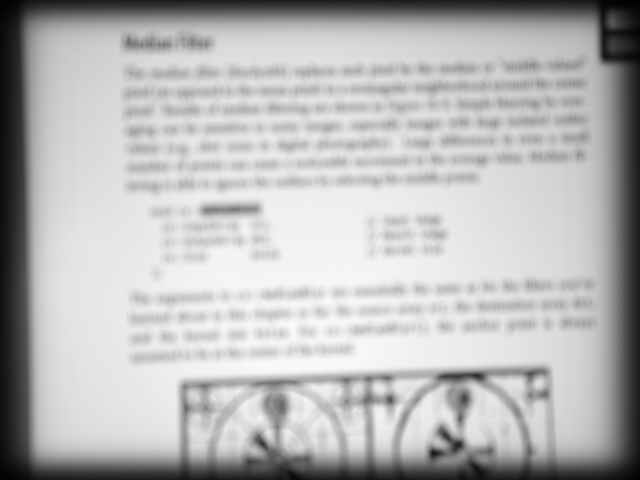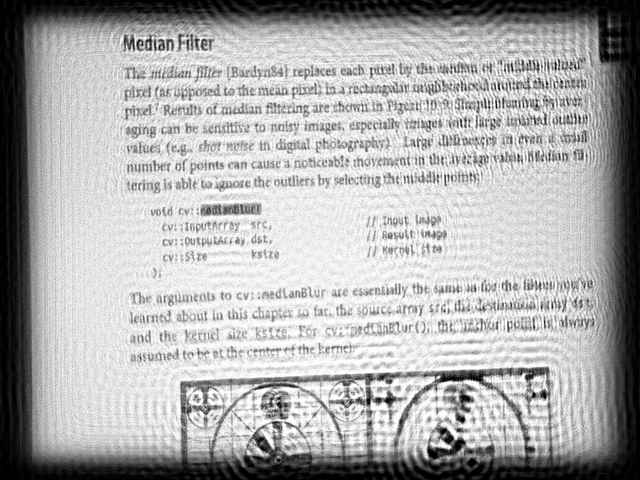OpenCV  4.6.0 Open Source Computer Vision
Out-of-focus Deblur Filter

Next Tutorial: Motion Deblur Filter

Compatibility OpenCV >= 3.0

## Goal

In this tutorial you will learn:

• what a degradation image model is
• what the PSF of an out-of-focus image is
• how to restore a blurred image
• what is a Wiener filter

## Theory

Note
The explanation is based on the books  and . Also, you can refer to Matlab's tutorial Image Deblurring in Matlab and the article SmartDeblur.
The out-of-focus image on this page is a real world image. The out-of-focus was achieved manually by camera optics.

### What is a degradation image model?

Here is a mathematical model of the image degradation in frequency domain representation:

$S = H\cdot U + N$

where $$S$$ is a spectrum of blurred (degraded) image, $$U$$ is a spectrum of original true (undegraded) image, $$H$$ is a frequency response of point spread function (PSF), $$N$$ is a spectrum of additive noise.

The circular PSF is a good approximation of out-of-focus distortion. Such a PSF is specified by only one parameter - radius $$R$$. Circular PSF is used in this work.### How to restore a blurred image?

The objective of restoration (deblurring) is to obtain an estimate of the original image. The restoration formula in frequency domain is:

$U' = H_w\cdot S$

where $$U'$$ is the spectrum of estimation of original image $$U$$, and $$H_w$$ is the restoration filter, for example, the Wiener filter.

### What is the Wiener filter?

The Wiener filter is a way to restore a blurred image. Let's suppose that the PSF is a real and symmetric signal, a power spectrum of the original true image and noise are not known, then a simplified Wiener formula is:

$H_w = \frac{H}{|H|^2+\frac{1}{SNR}}$

where $$SNR$$ is signal-to-noise ratio.

So, in order to recover an out-of-focus image by Wiener filter, it needs to know the $$SNR$$ and $$R$$ of the circular PSF.

## Source code

You can find source code in the samples/cpp/tutorial_code/ImgProc/out_of_focus_deblur_filter/out_of_focus_deblur_filter.cpp of the OpenCV source code library.

#include <iostream>
using namespace cv;
using namespace std;
void help();
void calcPSF(Mat& outputImg, Size filterSize, int R);
void fftshift(const Mat& inputImg, Mat& outputImg);
void filter2DFreq(const Mat& inputImg, Mat& outputImg, const Mat& H);
void calcWnrFilter(const Mat& input_h_PSF, Mat& output_G, double nsr);
const String keys =
"{help h usage ? | | print this message }"
"{image |original.JPG | input image name }"
"{SNR |5200 | signal to noise ratio}"
;
int main(int argc, char *argv[])
{
help();
CommandLineParser parser(argc, argv, keys);
if (parser.has("help"))
{
parser.printMessage();
return 0;
}
int R = parser.get<int>("R");
int snr = parser.get<int>("SNR");
string strInFileName = parser.get<String>("image");
if (!parser.check())
{
parser.printErrors();
return 0;
}
Mat imgIn;
if (imgIn.empty()) //check whether the image is loaded or not
{
cout << "ERROR : Image cannot be loaded..!!" << endl;
return -1;
}
Mat imgOut;
// it needs to process even image only
Rect roi = Rect(0, 0, imgIn.cols & -2, imgIn.rows & -2);
//Hw calculation (start)
Mat Hw, h;
calcPSF(h, roi.size(), R);
calcWnrFilter(h, Hw, 1.0 / double(snr));
//Hw calculation (stop)
// filtering (start)
filter2DFreq(imgIn(roi), imgOut, Hw);
// filtering (stop)
imgOut.convertTo(imgOut, CV_8U);
normalize(imgOut, imgOut, 0, 255, NORM_MINMAX);
imwrite("result.jpg", imgOut);
return 0;
}
void help()
{
cout << "2018-07-12" << endl;
cout << "DeBlur_v8" << endl;
cout << "You will learn how to recover an out-of-focus image by Wiener filter" << endl;
}
void calcPSF(Mat& outputImg, Size filterSize, int R)
{
Mat h(filterSize, CV_32F, Scalar(0));
Point point(filterSize.width / 2, filterSize.height / 2);
circle(h, point, R, 255, -1, 8);
Scalar summa = sum(h);
outputImg = h / summa;
}
void fftshift(const Mat& inputImg, Mat& outputImg)
{
outputImg = inputImg.clone();
int cx = outputImg.cols / 2;
int cy = outputImg.rows / 2;
Mat q0(outputImg, Rect(0, 0, cx, cy));
Mat q1(outputImg, Rect(cx, 0, cx, cy));
Mat q2(outputImg, Rect(0, cy, cx, cy));
Mat q3(outputImg, Rect(cx, cy, cx, cy));
Mat tmp;
q0.copyTo(tmp);
q3.copyTo(q0);
tmp.copyTo(q3);
q1.copyTo(tmp);
q2.copyTo(q1);
tmp.copyTo(q2);
}
void filter2DFreq(const Mat& inputImg, Mat& outputImg, const Mat& H)
{
Mat planes = { Mat_<float>(inputImg.clone()), Mat::zeros(inputImg.size(), CV_32F) };
Mat complexI;
merge(planes, 2, complexI);
dft(complexI, complexI, DFT_SCALE);
Mat planesH = { Mat_<float>(H.clone()), Mat::zeros(H.size(), CV_32F) };
Mat complexH;
merge(planesH, 2, complexH);
Mat complexIH;
mulSpectrums(complexI, complexH, complexIH, 0);
idft(complexIH, complexIH);
split(complexIH, planes);
outputImg = planes;
}
void calcWnrFilter(const Mat& input_h_PSF, Mat& output_G, double nsr)
{
Mat h_PSF_shifted;
fftshift(input_h_PSF, h_PSF_shifted);
Mat planes = { Mat_<float>(h_PSF_shifted.clone()), Mat::zeros(h_PSF_shifted.size(), CV_32F) };
Mat complexI;
merge(planes, 2, complexI);
dft(complexI, complexI);
split(complexI, planes);
Mat denom;
pow(abs(planes), 2, denom);
denom += nsr;
divide(planes, denom, output_G);
}

## Explanation

An out-of-focus image recovering algorithm consists of PSF generation, Wiener filter generation and filtering a blurred image in frequency domain:

// it needs to process even image only
Rect roi = Rect(0, 0, imgIn.cols & -2, imgIn.rows & -2);
//Hw calculation (start)
Mat Hw, h;
calcPSF(h, roi.size(), R);
calcWnrFilter(h, Hw, 1.0 / double(snr));
//Hw calculation (stop)
// filtering (start)
filter2DFreq(imgIn(roi), imgOut, Hw);
// filtering (stop)

A function calcPSF() forms a circular PSF according to input parameter radius $$R$$:

void calcPSF(Mat& outputImg, Size filterSize, int R)
{
Mat h(filterSize, CV_32F, Scalar(0));
Point point(filterSize.width / 2, filterSize.height / 2);
circle(h, point, R, 255, -1, 8);
Scalar summa = sum(h);
outputImg = h / summa;
}

A function calcWnrFilter() synthesizes the simplified Wiener filter $$H_w$$ according to the formula described above:

void calcWnrFilter(const Mat& input_h_PSF, Mat& output_G, double nsr)
{
Mat h_PSF_shifted;
fftshift(input_h_PSF, h_PSF_shifted);
Mat planes = { Mat_<float>(h_PSF_shifted.clone()), Mat::zeros(h_PSF_shifted.size(), CV_32F) };
Mat complexI;
merge(planes, 2, complexI);
dft(complexI, complexI);
split(complexI, planes);
Mat denom;
pow(abs(planes), 2, denom);
denom += nsr;
divide(planes, denom, output_G);
}

A function fftshift() rearranges the PSF. This code was just copied from the tutorial Discrete Fourier Transform:

void fftshift(const Mat& inputImg, Mat& outputImg)
{
outputImg = inputImg.clone();
int cx = outputImg.cols / 2;
int cy = outputImg.rows / 2;
Mat q0(outputImg, Rect(0, 0, cx, cy));
Mat q1(outputImg, Rect(cx, 0, cx, cy));
Mat q2(outputImg, Rect(0, cy, cx, cy));
Mat q3(outputImg, Rect(cx, cy, cx, cy));
Mat tmp;
q0.copyTo(tmp);
q3.copyTo(q0);
tmp.copyTo(q3);
q1.copyTo(tmp);
q2.copyTo(q1);
tmp.copyTo(q2);
}

A function filter2DFreq() filters the blurred image in the frequency domain:

void filter2DFreq(const Mat& inputImg, Mat& outputImg, const Mat& H)
{
Mat planes = { Mat_<float>(inputImg.clone()), Mat::zeros(inputImg.size(), CV_32F) };
Mat complexI;
merge(planes, 2, complexI);
dft(complexI, complexI, DFT_SCALE);
Mat planesH = { Mat_<float>(H.clone()), Mat::zeros(H.size(), CV_32F) };
Mat complexH;
merge(planesH, 2, complexH);
Mat complexIH;
mulSpectrums(complexI, complexH, complexIH, 0);
idft(complexIH, complexIH);
split(complexIH, planes);
outputImg = planes;
}

## Result

Below you can see the real out-of-focus image:Out-of-focus image

And the following result has been computed with $$R$$ = 53 and $$SNR$$ = 5200 parameters:The restored (deblurred) image

The Wiener filter was used, and values of $$R$$ and $$SNR$$ were selected manually to give the best possible visual result. We can see that the result is not perfect, but it gives us a hint to the image's content. With some difficulty, the text is readable.

Note
The parameter $$R$$ is the most important. So you should adjust $$R$$ first, then $$SNR$$.
Sometimes you can observe the ringing effect in a restored image. This effect can be reduced with several methods. For example, you can taper input image edges.

You can also find a quick video demonstration of this on YouTube.